# Pool coating

How many pieces of tiles 25 cm × 15 cm need to coat the bottom and side walls of the pool with bottom dimensions 30 m × 5 m, if the pool can fit up to 271500 liters of water?

Result

n =  7379

#### Solution:Leave us a comment of example and its solution (i.e. if it is still somewhat unclear...):

Showing 0 comments:Be the first to comment!## Next similar examples:

1. Soccer team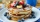Hilahs soccer team is trying to raise \$2414 to travel to a tournament in Florida, so they decided to host a pancake for the breakfast. How many people need to attend their breakfast in order to raise \$2414, if profit per one pancake is \$1.5?
2. Crates 2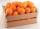One crate will hold 50 oranges. If Bob needs to ship 932 oranges, how many crates will he need?
3. Water reservoirThe water tank has a cuboid with edges a= 1 m, b=2 m , c = 1 m. Calculate how many centimeters of water level falls, if we fill fifteen 12 liters cans.
4. Hole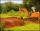They fill shape hole with dimensions 2.9 m, 17 m, 15.2 m with 97 m3 of soil. How many percent it fill up?
5. Fire tankHow deep is the fire tank with the dimensions of the bottom 7m and 12m, when filled with 420 m3 of water?
6. CuboidCuboid has a surface of 516 cm2. Side a = 6 cm and b = 12 cm. How long is the side c =?
7. Prism 4 sidesFind the surface area and volume four-sided prism high 10cm if its base is a rectangle measuring 8 cm and 1.2dm
8. Cuboid box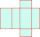Cuboid box have dimensions of 30 cm, 25 cm and 40 cm. Sketch its network and compute surface area of box.
9. CustomerA customer purchase three (3) writing pads from a store. She receive k9.70 change from a k10.00 note . How much was it's writing pad cost?
10. Cuboid - VabFind the surface of the cuboid when its volume is 52.8 cubic centimeters, and the length of its two edges is 2 centimeters and 6 centimeters.
11. Surface of cuboidFind the surface of the cuboid if its volume is 52.8 cm3 and the length of its two edges is 2 cm and 6 cm.
12. Cuboid - simple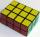Calculate the surface area and volume of a cuboid if a = 8 cm, b = 14 cm and c = 6 cm.
13. Cuboid surfaceDetermine surface area of cuboid if its volume is 52.8 cm cubic and length of the two edges are 2 cm and 6 cm.
14. Product of two fractionsProduct of two fractions is 9 3/5 . If one of the fraction is 9 3/7. Find the other fraction.
15. Cube surface areaWall of cube has content area 99 cm square. What is the surface of the cube?
16. Find xSolve: if 2(x-1)=14, then x= (solve an equation with one unknown)
17. Negative in equation2x + 3 + 7x = – 24, what is the value of x?